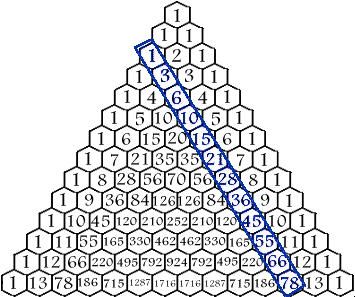In my last post, I didn’t explain the pattern to Jayson’s satisfaction and I had a typo in my proof that I have since corrected.

My proof demonstrated one pattern, namely that the square of an odd number minus one is divisible by eight. However, Jayson noticed that if you start with the first few odd numbers and go through those mathematical steps, the result of the operation leaves you with another series with interesting properties.

It turns out that series is the triangular series. I believe what Jayson wanted to know was why his function yielded this sequence. I shall dig into this here (notice I used the word shall? That’s a mathematician thang. You wouldn’t understand ;)) Here are the first few numbers in the sequence…

0, 1, 3, 6, 10,…

Another way to look at the series is…

` f(0) = 0 f(1) = 0 + 1 = 1 f(2) = 0 + 1 + 2 = 3 f(3) = 0 + 1 + 2 + 3 = 6 f(4) = 0 + 1 + 2 + 3 + 4 = 10 . . . f(n) = 0 + 1 + 2 + … + n - 1 + n = ???`

The n^th^ number in the series is the sum of all the numbers before n and including n. There’s a simple formula to get the n^th^ number in this series. Legend has it that Karl Friedrich Gauss discovered this while a very young student. He was told to sum up the numbers from 1 to 100 as a means to keep him busy for a long time. In a very short while, he came up with the answer. He observed that you could simply pair the numbers up like so…

` 1 + 100 = 101 2 + 99 = 101 3 + 98 = 101 . . . 50 + 51 = 101 = 50 * 101 = 5050`

It turns out, that the sum of all numbers n and below can be described by the simple formula…

`n(n+1)/2`

So how does this equation relate to the one Jayson showed us? Well to refresh your memory, that equation could be described as such

`f(xi) = (xi2 - 1)/8 = Ti`

In English, that means that applying his function to the i^th^ odd number yields the i^th^ triangular number.

So let’s start doing some simple algebraic substitutions. First, we need to define what we mean by the “i^th^” odd number. What is the odd number at i=0? Well that should clearly be the first odd number, one. So we state…

`xi = 2i + 1`

That’ll make sure we are only dealing with odd numbers. Now let’s substitute for x~i~

`f(xi) = f(2i + 1)`

Ok, this next step is a little tricky. By definition, f(x) = (x^2^ - 1)/8. This is Jayson’s formula. So let’s expand out f(2i + 1) into this formula. My assistant, the color green will assist to keep this clear.

`f(xi) = ((2i + 1)2 - 1)/8`

By now, I am really wishing HTML supported math symbols easily. Now doing some multiplying.

`f(xi) = (4i2 + 4i + 1 - 1)/8`

Doing a bit of arithmetic leads us to

`f(xi) = (4i2 + 4i)/8`

Some factorization…

`f(xi) = 4i(i + 1)/8`

Doing some division (man this math stuff is hard)

`f(xi) = i(i + 1)/2`

Does that look familiar? I hope you are having an aha moment (if you didn’t have it a long time ago). That is the formula for the i^th^ triangular number! Thus with a bit of algebra, I have demonstrated that…

`f(xi) = (xi2 - 1)/8 = i(i + 1)/2 = Ti`

So that is why his function reveals the triangular number series.

One interesting thing about triangular numbers are their connection to Pascal’s triangle as evident in this image I found at this site.Trippy eh? You gotta love the various diversions mathematicians come up with to keep themselves busy.